# 8kN/m5 kN/m-0.75 m---- 0.75 m-1.5 m-

Question

Replace the loading by a single resultant force, specify the location of force measured from point O.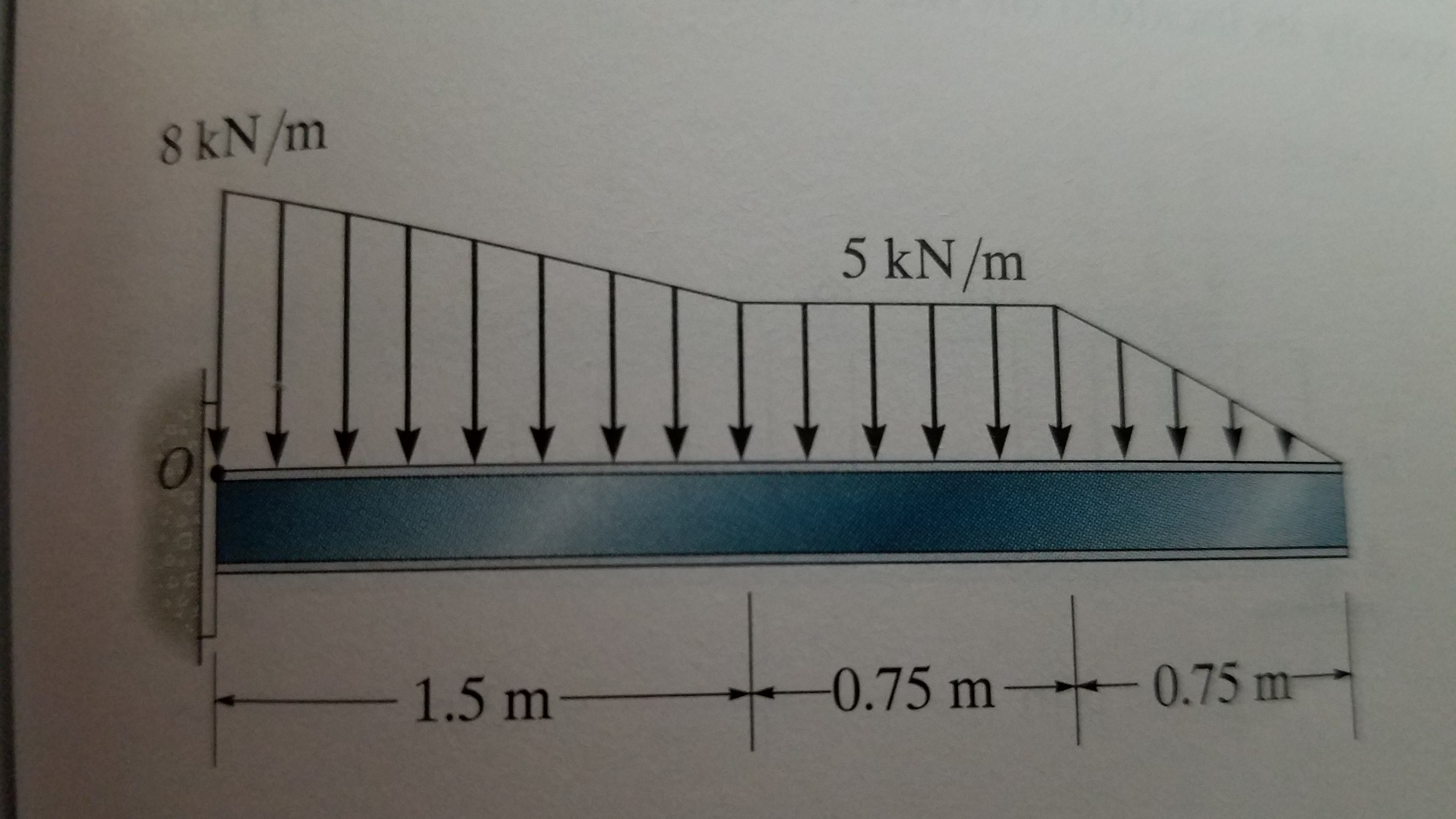help_outlineImage Transcriptionclose8kN/m 5 kN/m -0.75 m---- 0.75 m -1.5 m- fullscreen
check_circleExpert Solution
Step 1
Step 2

The area of the triangle NBC is equal to the magnitude of the single point load. Thus,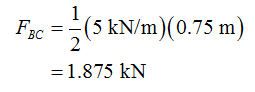The position of the single point load of section BC is equal to the 1/3rd from the base. Thus,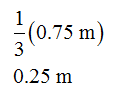From the point O, the position of the point load is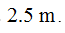Step 3

The area of the triangle MNBC is equal to the magnitude of the single point load. Thus,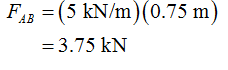The position of the single point load for section AB is equal to the 1/2rd from the base. Thus,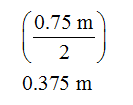From the point O, the position of the point load is 1.875 m.

...

### Want to see the full answer?

See Solution

#### Want to see this answer and more?

Solutions are written by subject experts who are available 24/7. Questions are typically answered within 1 hour*

See Solution
*Response times may vary by subject and question
Tagged in

### Mechanical Engineering